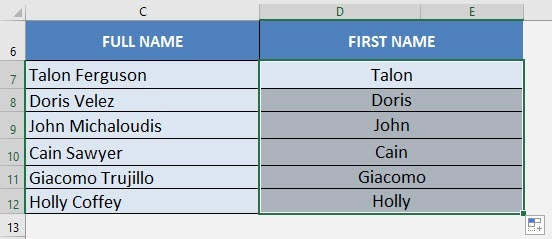There were countless times when I had a list of full names, and all I needed was the First Name. It would be time-consuming to manually get the first names one by one. Thank goodness there are formulas to make my life easier!

In Excel, it’s very easy to do that with the LEFT and FIND formula!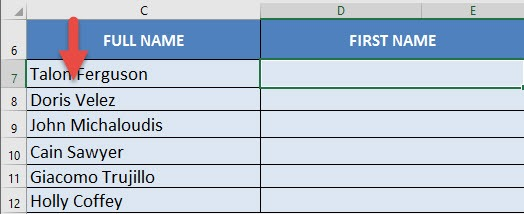Here is the gameplan:

• Use the FIND formula to find the location of the space that separates the First Name and the Last Name
• However we need to deduct this numerical location by 1, so that we have the location of the end of the First Name
• With this number, we will use the LEFT formula to retrieve the First Name!

I explain how you can do this below:

STEP 1: We need to enter the LEFT function and select the Full Name:

## =LEFT(C7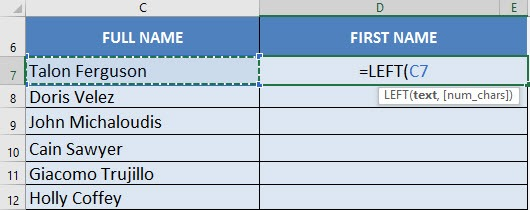STEP 2: We need to enter the FIND formula to get the empty space location between the first and last name:

## =LEFT(C7, FIND(” “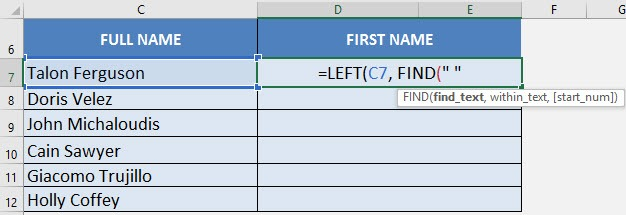STEP 3: Select the Full Name again for the FIND formula’s 2nd argument:

## =LEFT(C7, FIND(” “, C7)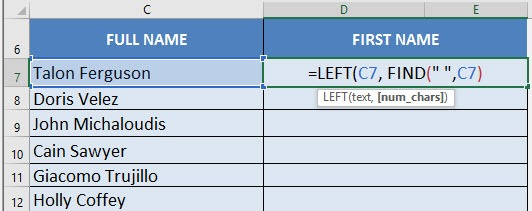STEP 4: Deduct 1 from the FIND formula so that our result will return us the text up to the last letter of the first name:

## =LEFT(C7, FIND(” “, C7) -1)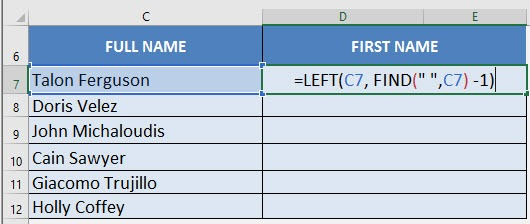STEP 5: Do the same for the rest of the cells by dragging the formula all the way down using the left mouse button.

Now you are able to extract all the First Names from your FULL NAME using the FIND formula in Excel!